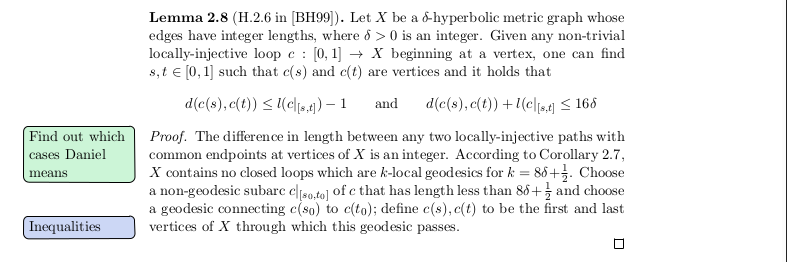LaTeX todonotes

Currently I'm writing my bachelor thesis in mathematics and for noting what I still have to do or mark some difficulties, I used to add comments to the .tex-code. However, when printing the file or viewing the compiled output, these obviously won't be displayed. Gladly a quick search for latex todo yielded todonotes, a simple to use package which puts todo notes to the margin of the document.

The usage is quite simple; if it's installed, just add \usepackage{todonotes} to your preamble and you can simply put in todo notes by usage of \todo{Something I have to do}, and then they magically appear at this position on the margin of your page. At the moment I only need two different kinds of todo notes, so I added the following lines:

\usepackage{todonotes}
\setlength{\marginparwidth}{3cm} % make todonotes appear wider
\reversemarginpar % make todonotes appear on the left

\newcommand{\tproof}{\todo[color=blue!80!green!20,noline]{#1}}
\newcommand{\tdo}{\todo[color=blue!20!green!20,noline]{#1}}

As you can see, I modified the width of the todo notes, since they were a bit too small for longer words, and I set them to appear on the left instead of the right. Finally I created two new commands, \tproof and \tdo, one for things I have to proof and one for general thoughts. Here is an example of the usage:

\begin{proof}
\tdo{Find out which cases Daniel means}
The difference in length between any two locally-injective paths with common
endpoints at vertices of $X$ is an integer. According to
\cref{no closed loops},
$X$ contains no closed loops which are $k$-local geodesics for $k = 8\delta + \frac{1}{2}$. Choose a non-geodesic subarc $c|_{[s_0,t_0]}$ of $c$ that
has length less than $8\delta+\frac{1}{2}$ and choose a geodesic connecting
$c(s_0)$ to $c(t_0)$; define $c(s),c(t)$ to be the first and last vertices
of $X$ through which this geodesic passes.
\tproof{Inequalities}
\end{proof}

And the resulting output:I recommend to read the comprehensive documentation of todonotes since the package has some other nice features, like adding the option to insert placeholders for figures or only showing the todo notes when in draft mode.